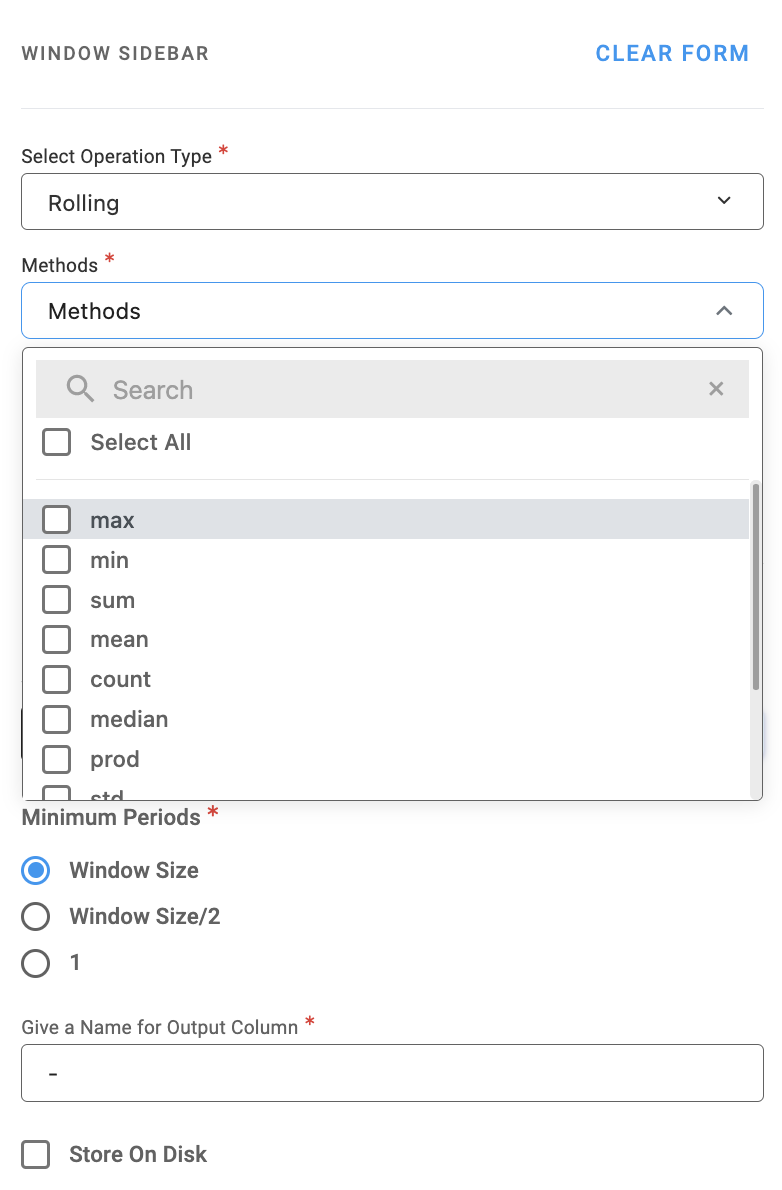# Window

Compute rolling or expanding on a window of rows in your dataset.

A rolling function, also known as a moving average or a rolling window function, is commonly used in time series data analysis to smooth out short-term fluctuations and highlight longer-term trends or cycles.

An expanding function, also known as an expanding window function, is a technique used in time series data analysis to calculate a statistic over a window that expands over time. This means that, for each data point, the statistic is computed over all previous data points up to and including the current data point. A common use case for an expanding function is to compute the cumulative sum, mean, or other statistics of a time series.Example

Dataset:

A
3
5
8
6
9
11
12
15
7
4

Result: (Window Size: 3)

B
NaN
NaN
5.333333333
6.333333333
7.666666667
8.666666667
10.66666667
12.66666667
11.33333333
8.666666667